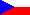Ceska verze teto stranky Back to main page | list of seminars

### BE2M31ZRE seminar Recognition of vowel sequence based on HMM - part II

Definition and construction of HMM model
Computation of emitted probabilities and passing likelihood through whole HMM model
Occupacy likelihood of particular states of HMM (Backward Viterbi algorithm)

• Definition of HMM-model transient matrix, computation of emitted probabilities in HMM states
• Determine parameters of HMM transient matrix for the supposed utterance in the following form - duration of each vowel same as separation pause is 1s. Save parameters into the structure variable hmm.a in the related HMM model. Order of the matrix A should be hmm.states.
• Compute the matrix of all emitted log-probabilities (with suitable thresholding) for all states of given HMM model. This matrix should have the number of rows related to the number of states in HMM model and number of columns related to the number of feature vectors (short-time frames) in analyzed utterance.
• Result: Display for the utternaces P1, P2 and P3 :
• Computed emitted log-probabilities for all states (use function pcolor followed by shading interp).
• Display in command prompt (or variable space) matrix of transient probabilities A.

• Computation of likelihood of passing through HMM using Viterbi algorithm
• Create function myviterbi for the computation of likelihood of passing through HMM model based on Viterbi algorithm. Input of this function should be matrix of feature vectors (cepstra) and chosen HMM model. Output should be the final likelihood of passing through HMM model.
• Checked result (3 points): Display for test utterances containing sequences of vowels:

• Determine optimum path using backtracing of passing through HMM model.
• Determine boundaries of particular vowels in utterances P1, P2 and P3.
• Optional result (BONUS 1 point): Display for test utterances P1, P2 and P3
• Display also the line representing the optimum path into the plot with emitted probabilities in all states (previous checked result).
• Waveform and spectrogram with determined phone boudaries for correct utterance P1.

• On the basis of known boundaries, precise transient matrix A and compute on more time likelihoods of passing through HMM model with corrected transient probabilities.

• Occupacy likelihood of particular states of HMM
• In prepared function myviterbi save also the matrix of all forward probabilities alpha related to each state and time during the passing through HMM model.
• Create also the functions mybackviterbi (analogous to myviterbi) which realizes backward computation of passing likelihood through HMM (i.e. from the last state to the first one using probabilities beta). Take as an input of this function again the matrix of feature vectors nad given HMM model. The output should be global probability of passing through the model same as the matrix of all backward probabilities beta for each state and each time.
• Optional result (BONUS 1 point): display for utterances P1, P2 and P3
• computed forward and backward probabilities of passing through HMM model for the utternace AEIOU,
• occupacy likelihood for all states and all times (attention, it is necessary to use same colorscaling when occupaccy likelihood is presented for different utterances P1, P2 and P3.

• Both BONUS results should be delivered within the previous solution of basic Viterbi decoding and the same above mentioned WEB interface.

• Try also the computation of probabilities using standard forward or backward procedure respectively.